# BGN-1.1.1

Note that the definition of trapezoid and trapezium here accord to the American definition.

# Formulae 公式

## triangles 三角形

Representation of symbols—Image 1.1.1-1

Area 面积:
\begin{aligned} S&=\frac{1}{2}bh\\ &=ab\sin C\\ &=\sqrt{s(s-a)(s-b)(s-c)(s-d)} \\ &=\frac{abc}{4R}\\ &=\frac{1}{2}r(a+b+c) \end{aligned} The third line is Heron’s formula, r & R are the in-radius and circumradius of the triangle.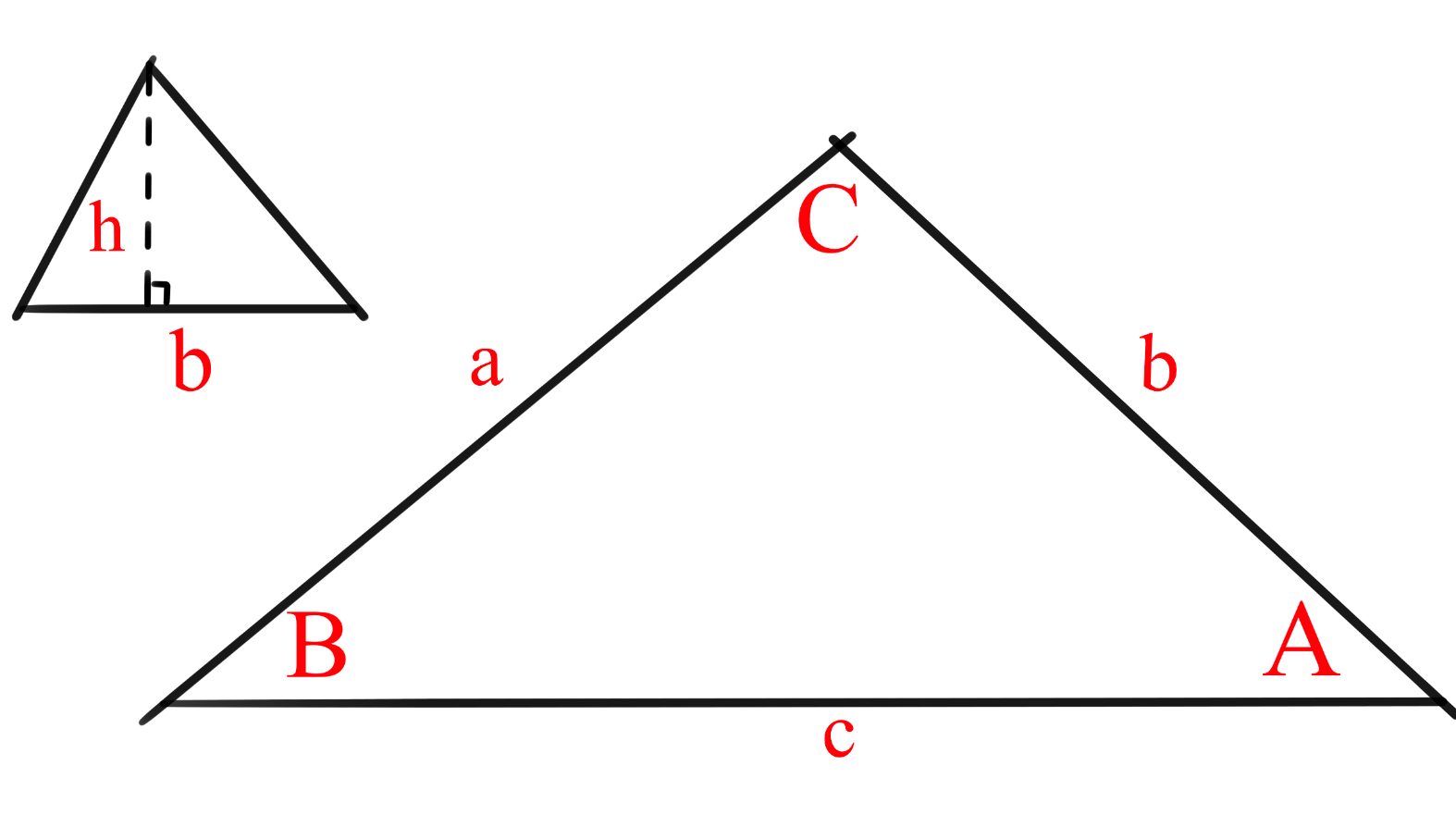Image 1.1.1-1 图1.1.1-1

Representation of symbols—Image 1.1.1-2

Square 正方形: $s^2$
Rectangle 長方形: $ab$
Trapezoid 梯形: $\dfrac{1}{2} (a+b)h$
Parallelogram 平行四边形: $bh$, $ab\sin \theta$
Rhombus 菱形: $\dfrac{1}{2} cd$, $s^2\sin \theta$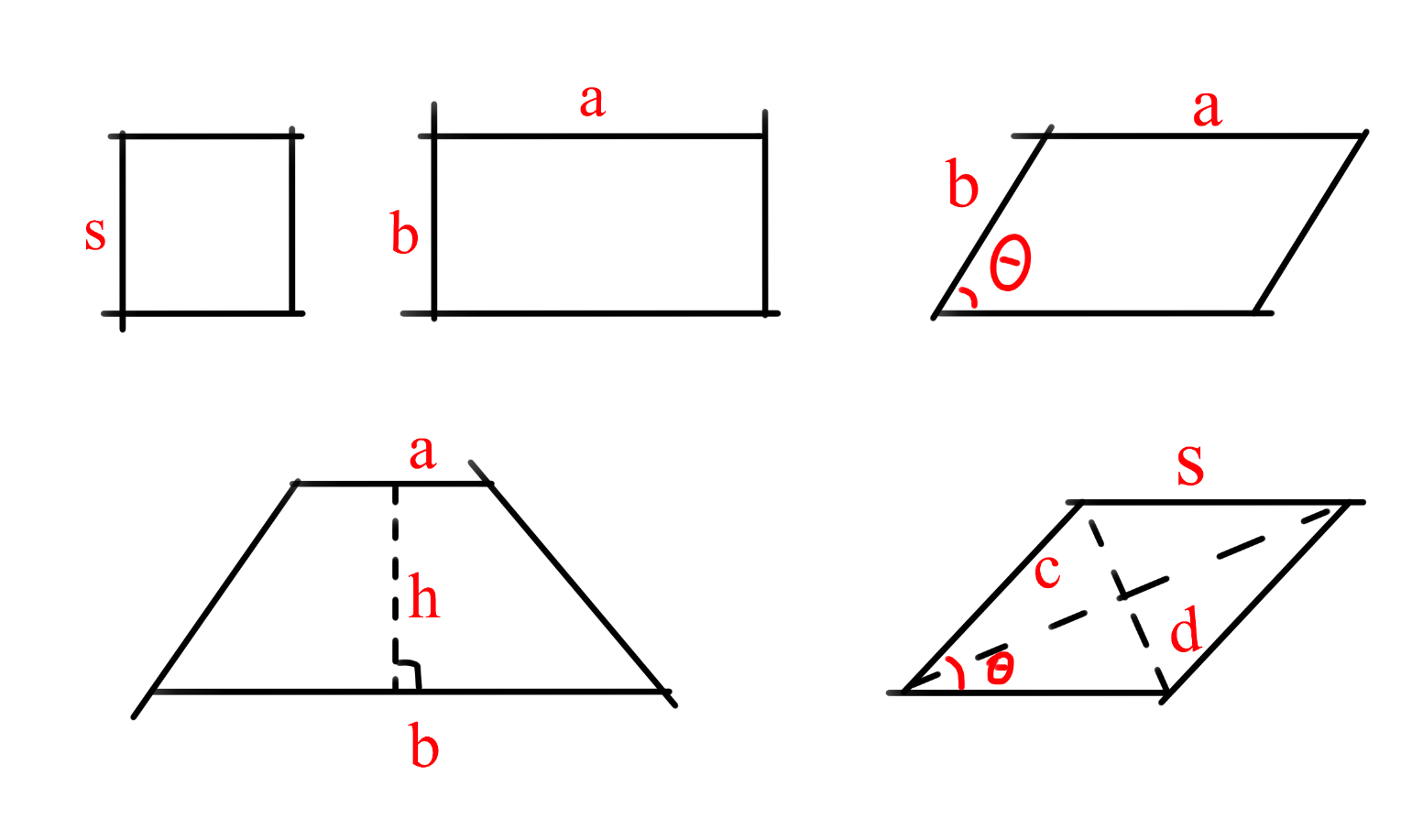Image 1.1.1-2 图1.1.1-2 P.S. The area of a unit rhombus is equal to $\sin$ $\theta$ and $\angle \theta$ is acute (smaller than $90^\circ$).

## ellipticals 椭圆形

Circle 圆: $\pi r^2$, where $r$ is the radius. $\pi r^2$$r$ 代表半径。
Oval 椭圆: $\pi ab$, where $a$ is half the long axis, $b$ is half the short axis. $\pi ab$$a,b$ 分别指长轴的一半和短轴的一半。

# Proof 证明

Imagine a rectangle grid (chocolate bar) with $a$ rows and $b$ columns of unit grids (squares of side length 1 unit). It will have $a$ rows of length $b$, i.e. $a$ rows of area $b$, so it’s size is $\underset{\text{a times}}{\underbrace{b+b+...+b}}=ab.$ Same for squares.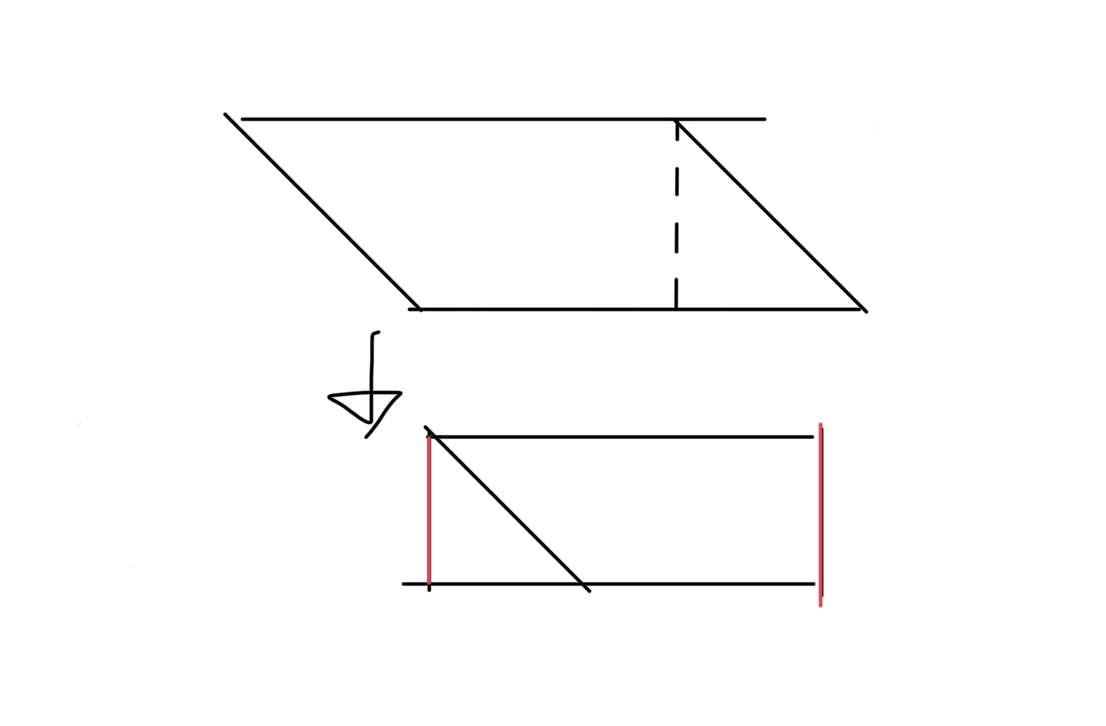Image 1.1.1-3 图1.1.1-3
As for parallelograms (including rhombuses), we can split them into two like this $\rightarrow$
so it’s area is $\dfrac{1}{2} bh$.

Two identical trapezoids can be put together to form a parallelogram with base $a+b$, height $h$, so one trapezoid has size $\dfrac{1}{2} (a+b)h$.

The other formulae of quadrilaterals mentioned above will not be specifically explained, but you can check the wiki and solve yourself. 其他四边形的上述公式不在此详解，如有兴趣可自行推导。

## triangles 三角形

Most triangle size formulae are given by half the size formulae of parallelograms.

## ellipticals 椭圆形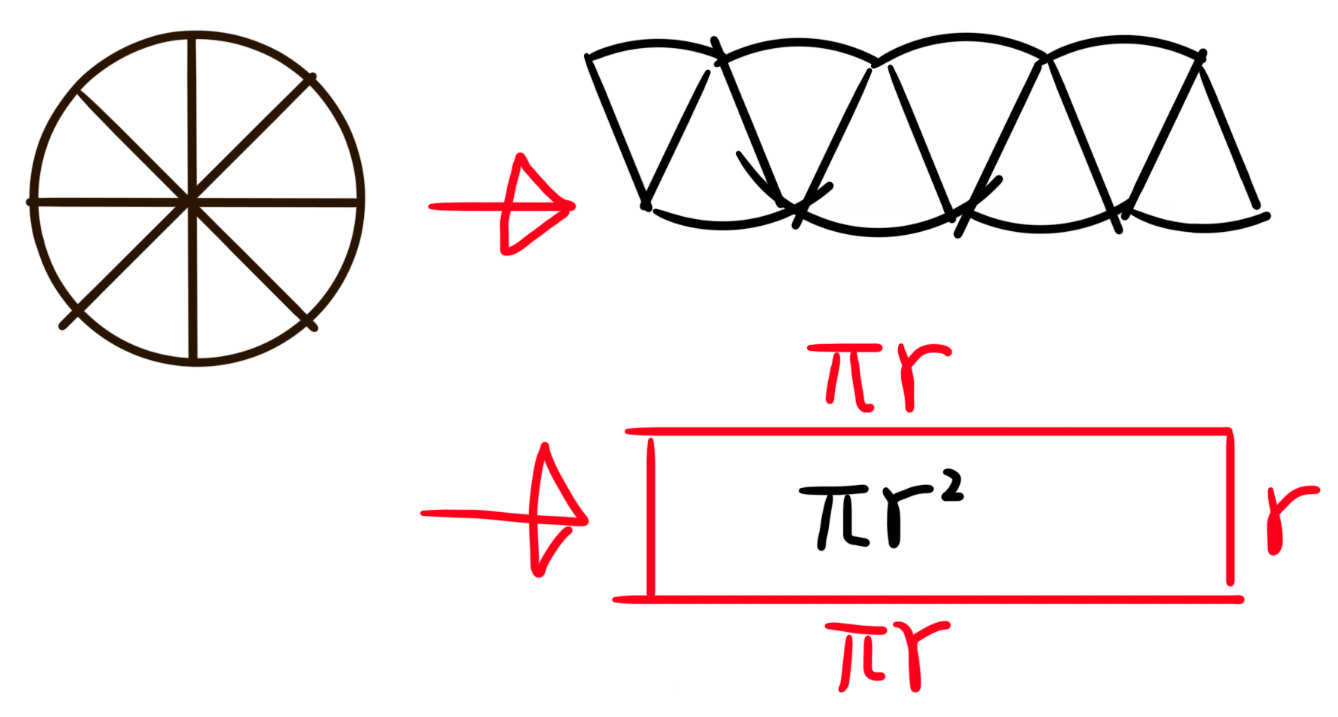Image 1.1.1-4 图1.1.1-4 Split a circle into equal pieces. Arranging, we get a ‘rectangle’. The more pieces the circle is split in, the more close the arranged shape is to a rectangle with base $\pi r$, height $r$.

# Techniques 技巧

For other polygons, you can either split them up or think of them as a few small polygons taken from a big one.Note by Jeff Giff
3 months, 4 weeks ago

This discussion board is a place to discuss our Daily Challenges and the math and science related to those challenges. Explanations are more than just a solution — they should explain the steps and thinking strategies that you used to obtain the solution. Comments should further the discussion of math and science.

When posting on Brilliant:

• Use the emojis to react to an explanation, whether you're congratulating a job well done , or just really confused .
• Ask specific questions about the challenge or the steps in somebody's explanation. Well-posed questions can add a lot to the discussion, but posting "I don't understand!" doesn't help anyone.
• Try to contribute something new to the discussion, whether it is an extension, generalization or other idea related to the challenge.

MarkdownAppears as
*italics* or _italics_ italics
**bold** or __bold__ bold
- bulleted- list
• bulleted
• list
1. numbered2. list
1. numbered
2. list
Note: you must add a full line of space before and after lists for them to show up correctly
paragraph 1paragraph 2

paragraph 1

paragraph 2

[example link](https://brilliant.org)example link
> This is a quote
This is a quote
    # I indented these lines
# 4 spaces, and now they show
# up as a code block.

print "hello world"
# I indented these lines
# 4 spaces, and now they show
# up as a code block.

print "hello world"
MathAppears as
Remember to wrap math in $$ ... $$ or $ ... $ to ensure proper formatting.
2 \times 3 $2 \times 3$
2^{34} $2^{34}$
a_{i-1} $a_{i-1}$
\frac{2}{3} $\frac{2}{3}$
\sqrt{2} $\sqrt{2}$
\sum_{i=1}^3 $\sum_{i=1}^3$
\sin \theta $\sin \theta$
\boxed{123} $\boxed{123}$

Sort by:

Report to moderators: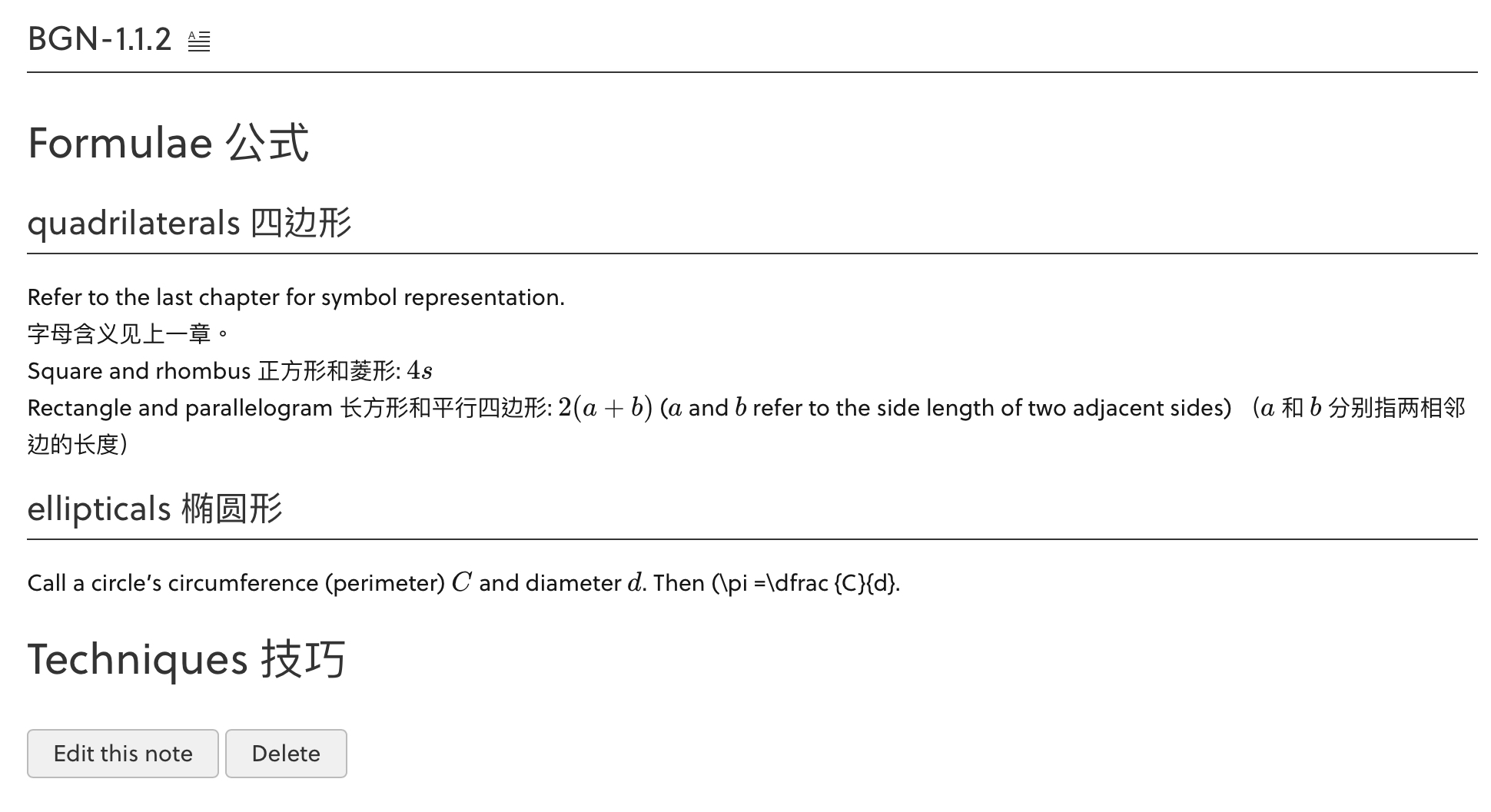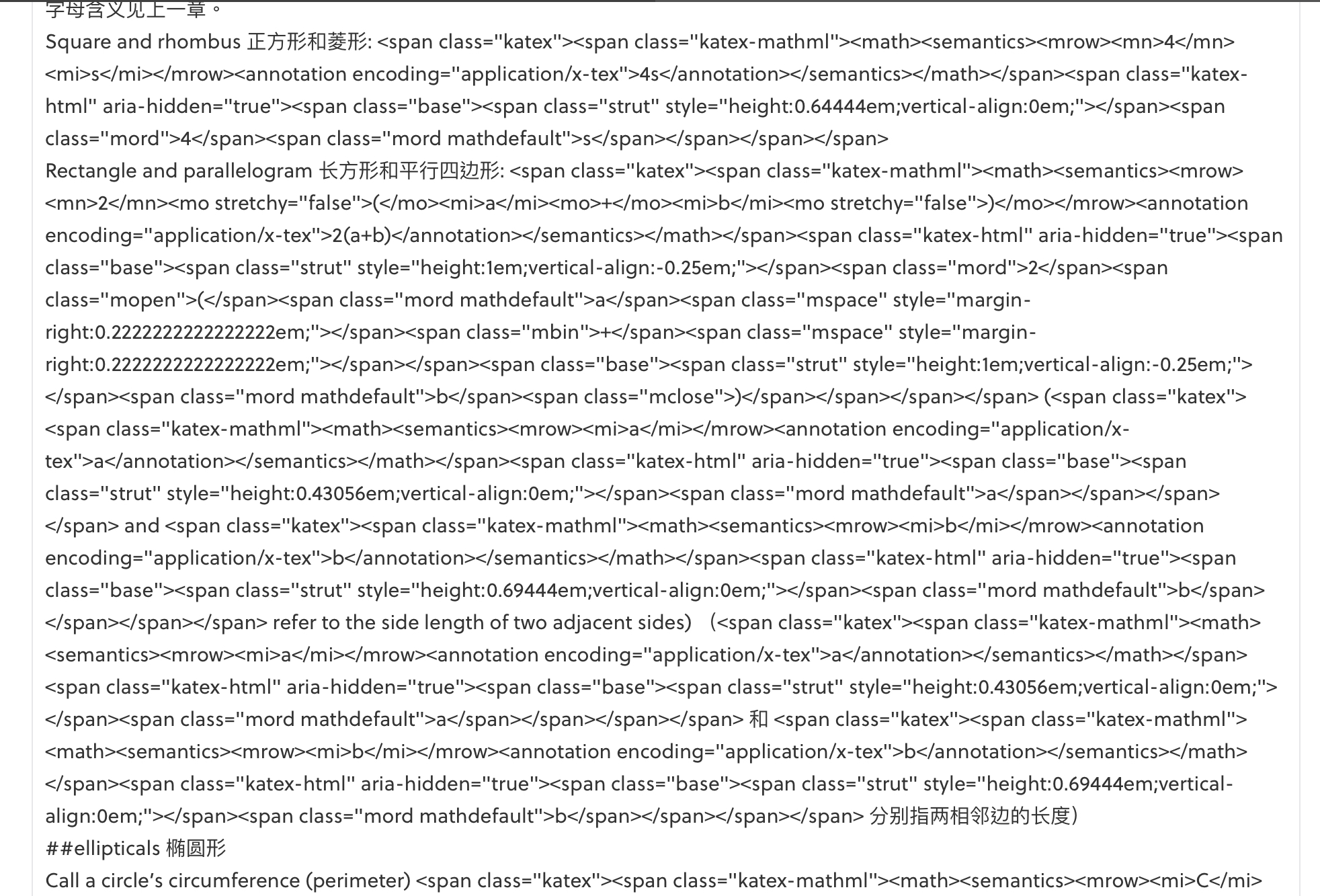This happened when I tried to edit my OTHER note but forgot to close a LaTeX bracket. Then the note malfunctioned and no matter how I refreshed my browser it still showed up wrong. :( Please help! 🙏
@Brilliant Mathematics

- 3 months, 3 weeks ago

Hi Jeff, this page looks fine by me. Do you still face the same issue?

Staff - 3 months, 3 weeks ago

THIS page is fine, but it’s my OTHER page that malfunctioned :)

- 3 months, 2 weeks ago

Hi Jeff, the other pages look fine by me as well.

Please send me the relevant URL of your notes that have been affected. Thank you.

Staff - 3 months, 2 weeks ago

I know:

- 3 months, 2 weeks ago

- 3 months, 3 weeks ago

Put this one and the Bilingual Geometry Notebook in your RadMaths note!

- 3 months, 4 weeks ago

Yup, working on that

- 3 months, 4 weeks ago